0

# 10个实用的数据可视化的图表总结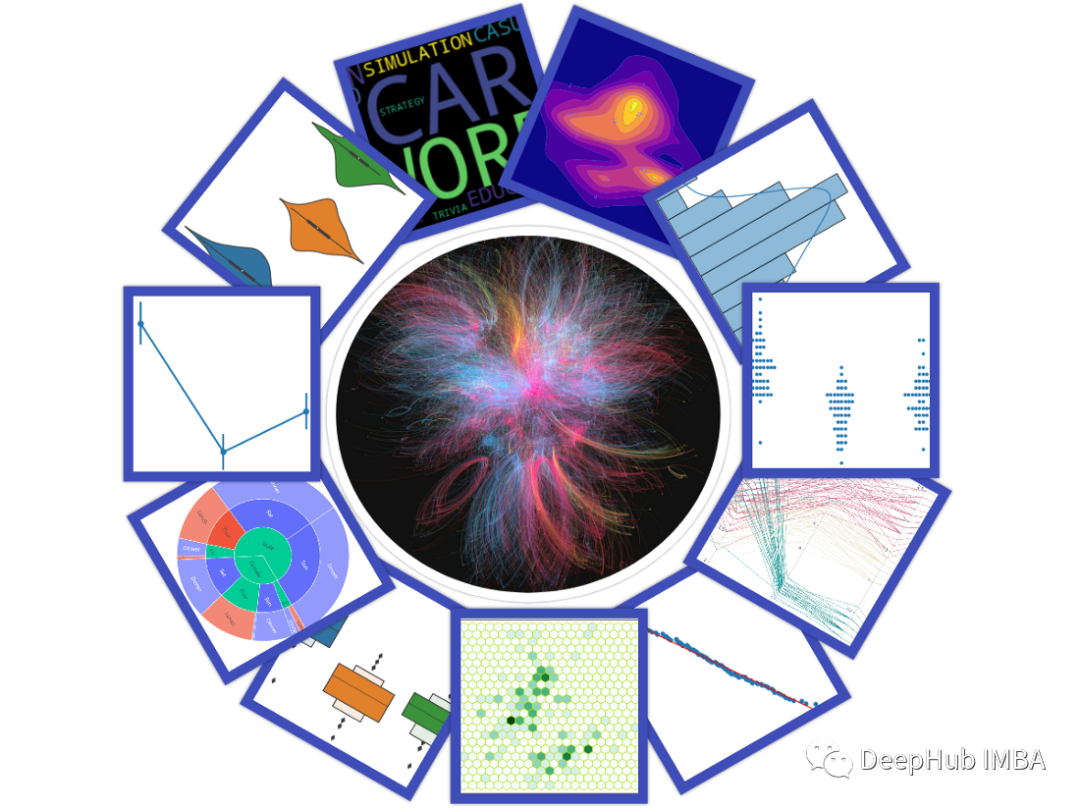## 1、平行坐标图（Parallel Coordinate）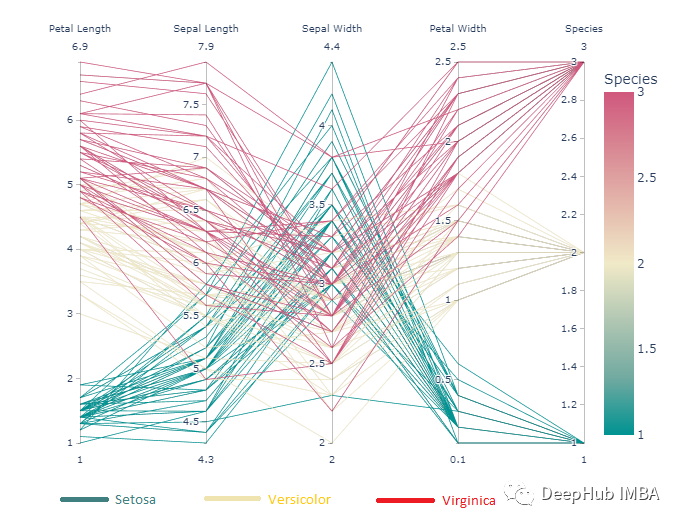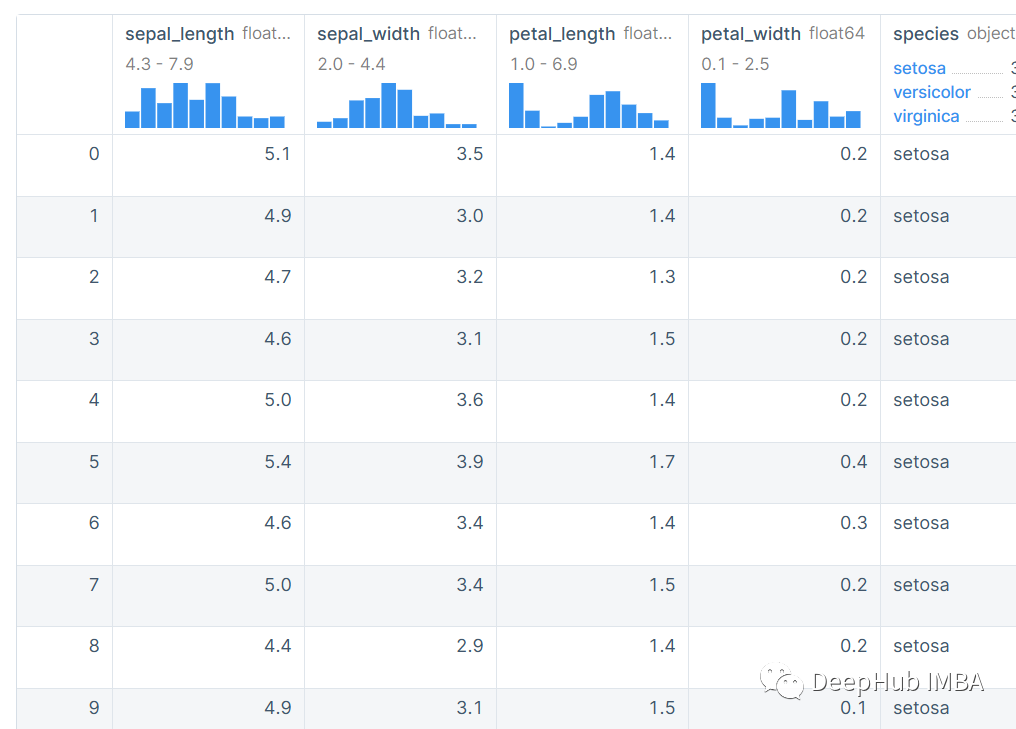`````` import plotly.express as px
df = px.data.iris()
fig = px.parallel_coordinates(df, color="species_id", labels={"species_id": "Species",
"sepal_width": "Sepal Width", "sepal_length": "Sepal Length",
"petal_width": "Petal Width", "petal_length": "Petal Length", },
color_continuous_scale=px.colors.diverging.Tealrose,
color_continuous_midpoint=2)
fig.show()
``````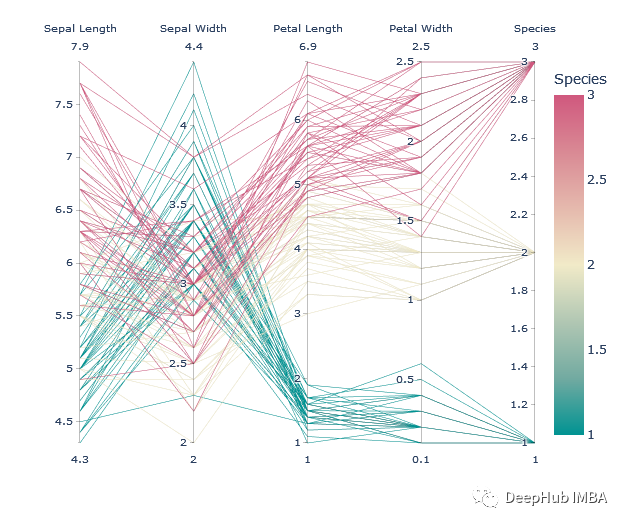## 2、六边形分箱图 （Hexagonal Binning）

`````` ax = df.plot.hexbin(x='sepal_width', y='sepal_length', gridsize=20,color='#BDE320')
``````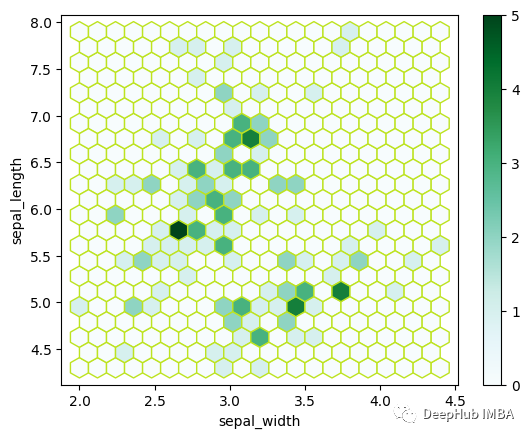## 3、等高线密度图（Contour ）

`````` import plotly.express as px
fig = px.density_contour(df, x="sepal_width", y="sepal_length")
fig.update_traces(contours_coloring="fill", contours_showlabels = True)
fig.show()
``````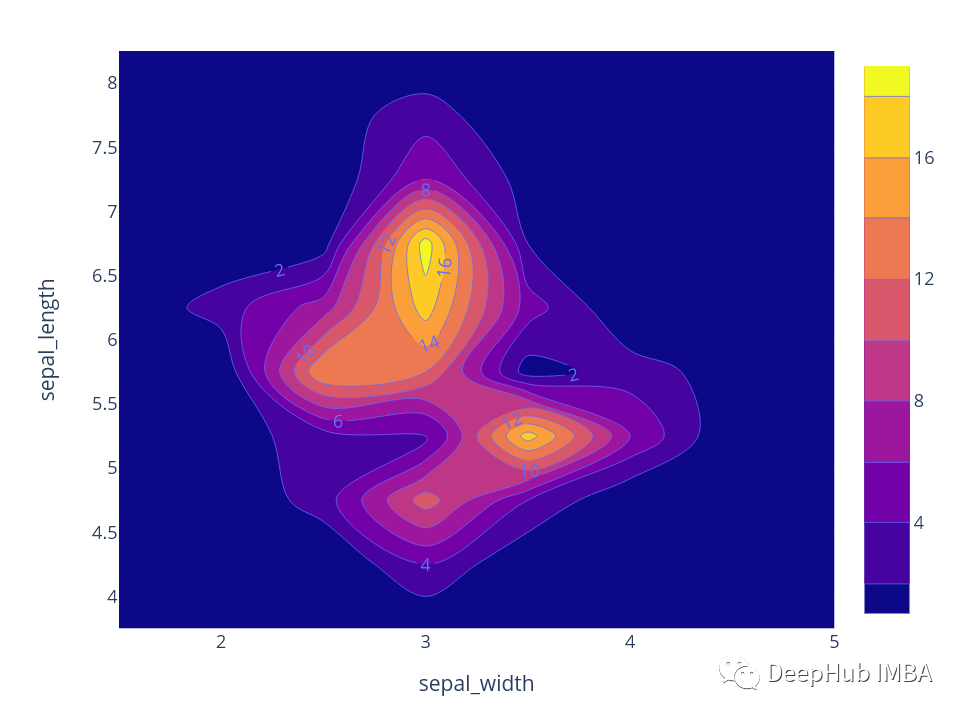## 4、QQ-plot

QQ plot是另一个有趣的图。QQ是Quantile - Quantile plot的缩写（Quantile/percentile是一个范围，在这个范围内数据下降了指定百分比。例如，第10个quantile/percentile表示在该范围下，找到了10%的数据，90% 超出范围）。这是一种直观地检查数值变量是否服从正态分布的方法。让我解释一下它是如何工作的。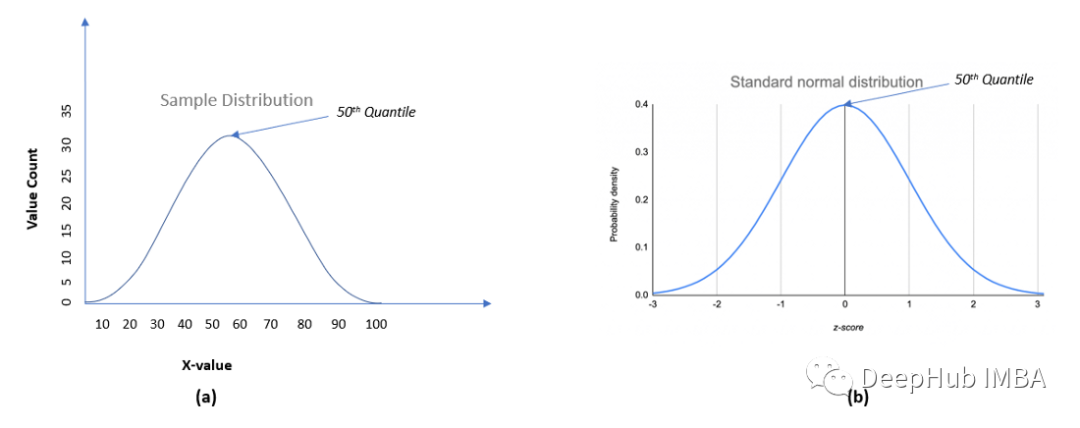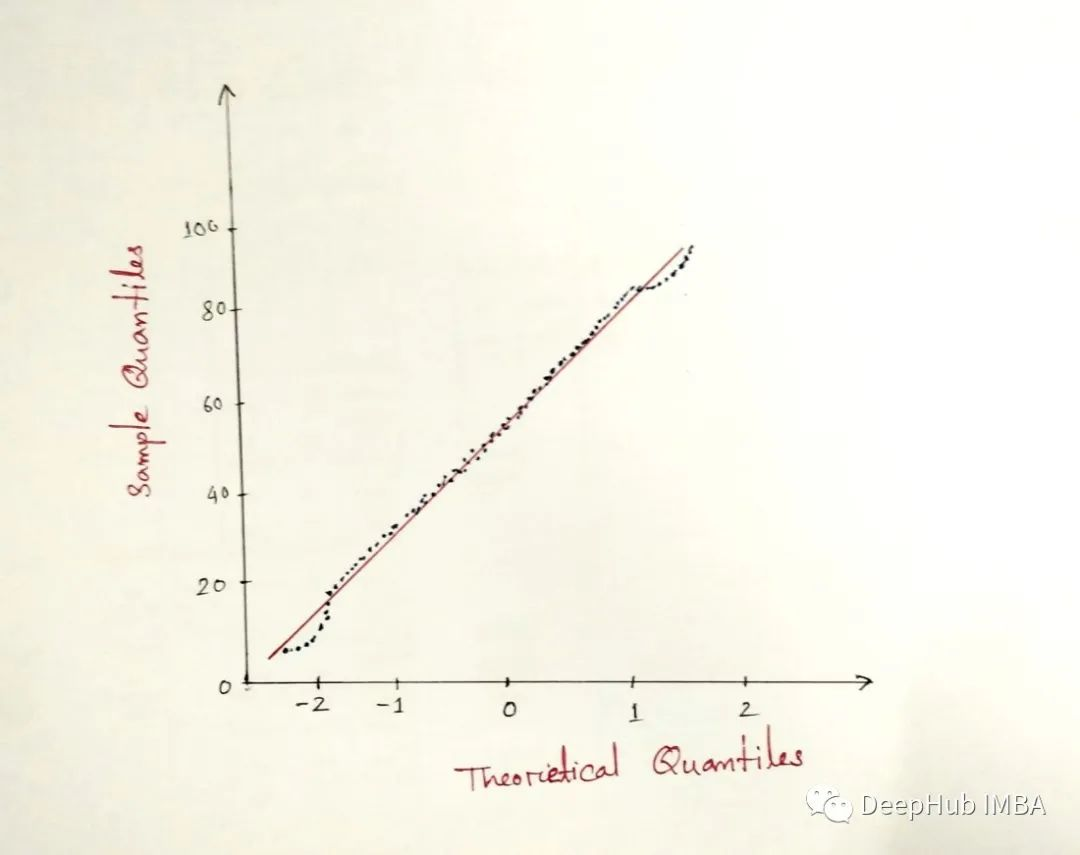`````` import pandas as pd
import numpy as np
import matplotlib.pyplot as plt
import seaborn as sns
``````

`````` np.random.seed(10)
# Generate Univariate Observations
gauss_data = 5 * np.random.randn(100) + 50
``````

`````` sns.histplot(data=gauss_data, kde=True)
``````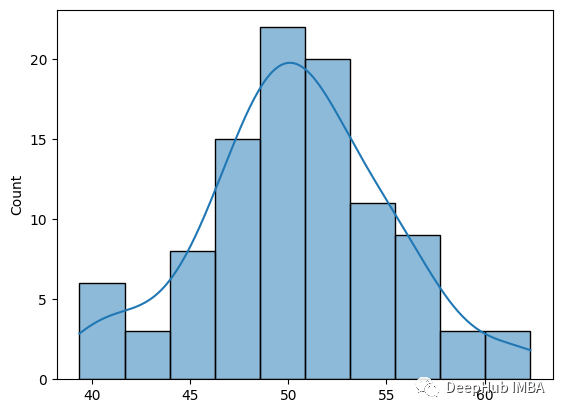`````` import statsmodels.api as sm
# q-q plot
sm.qqplot(gauss_data, line='s')
plt.show()
``````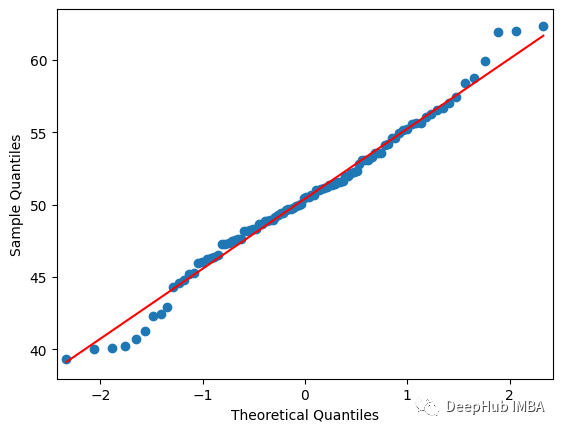## 5、小提琴图(Violin Plot)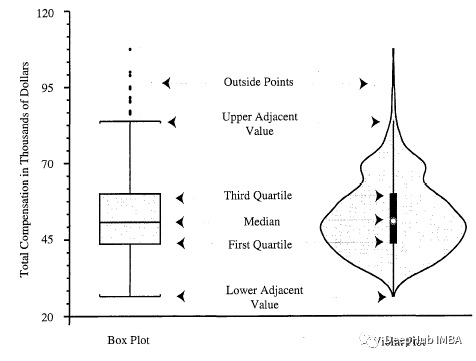`````` import seaborn as sns
sns.violinplot(data=df, y="sepal_width")
``````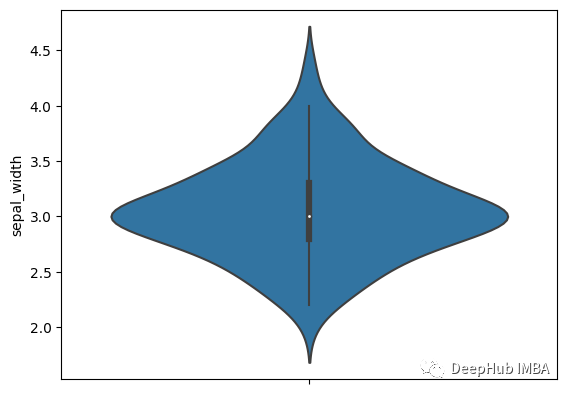`````` import seaborn as sns
sns.violinplot(data=df,x='species', y="sepal_width")
``````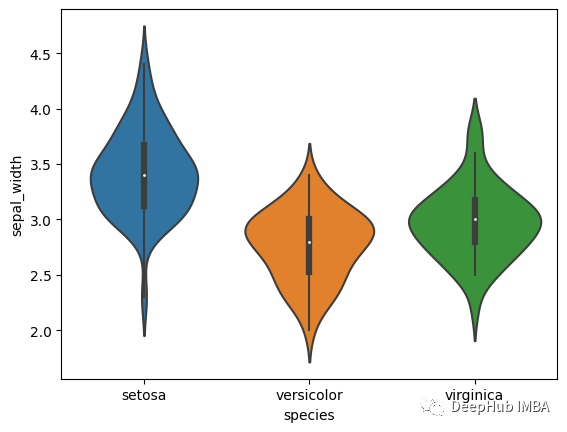## 6、箱线图的改进版(Boxen plot)

Boxenplot 是 seaborn 库引入的一种新型箱线图。对于箱线图，框是在四分位数上创建的。但在 Boxenplot 中，数据被分成更多的分位数。它提供了对数据的更多内存。

`````` sns.boxenplot(x=df["sepal_width"])
``````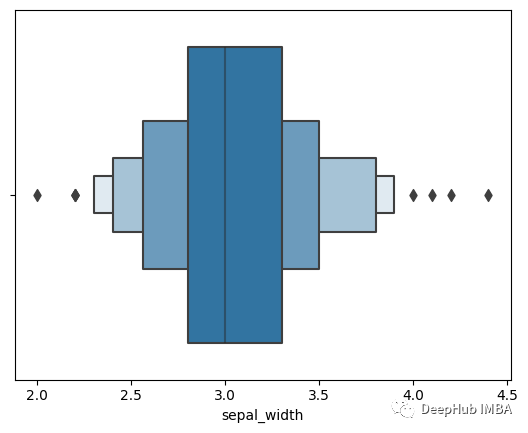`````` sns.boxenplot(data=df, x="species",y='sepal_width')
``````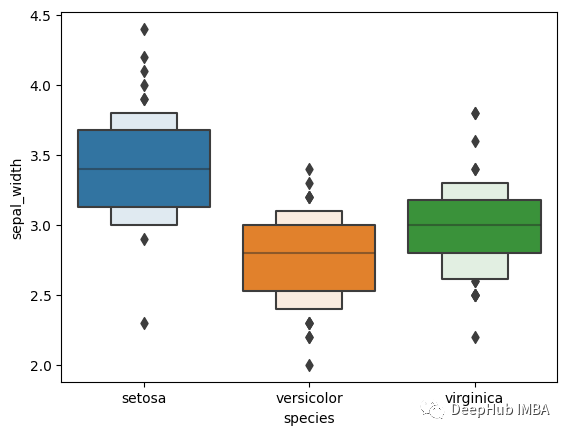## 7、点图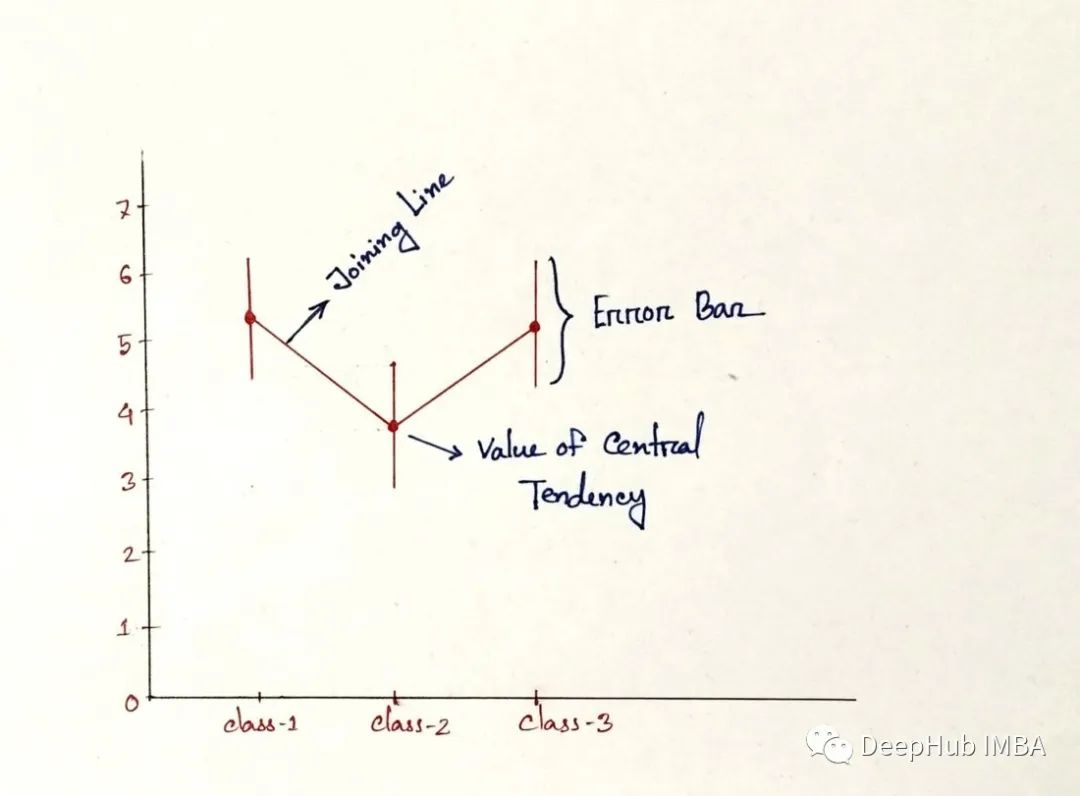`````` import seaborn as sns
sns.pointplot(data=df,x="species", y="sepal_width")
``````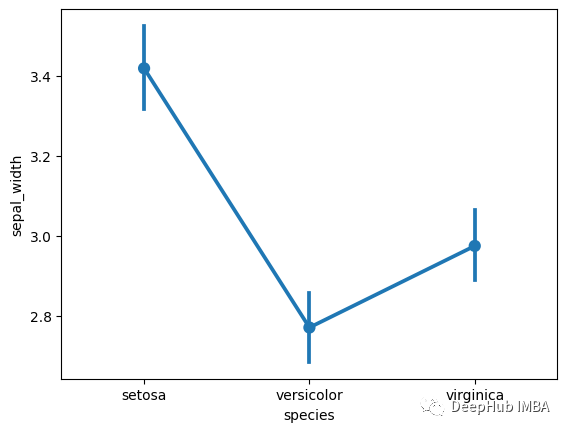## 8、分簇散点图（Swarm plot）

Swarm plot 是另一个受“beeswarm”启发的有趣图表。通过此图我们可以轻松了解不同的分类值如何沿数值轴分布 。它在不重叠数据点的情况下绘制数据。但它不适用于大型数据集。

`````` import seaborn as sns
sns.swarmplot(data=df,x="species", y="sepal_width")
``````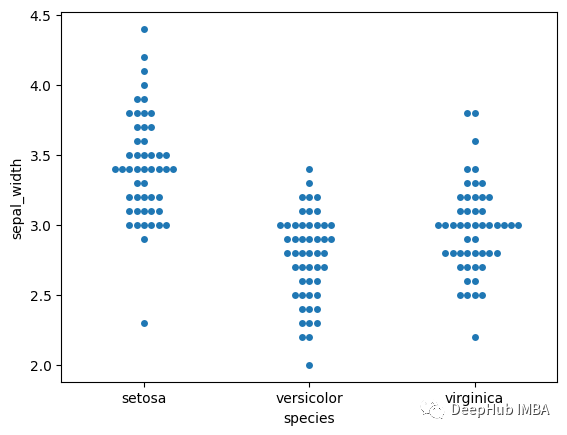## 9、旭日图（Sunburst Chart）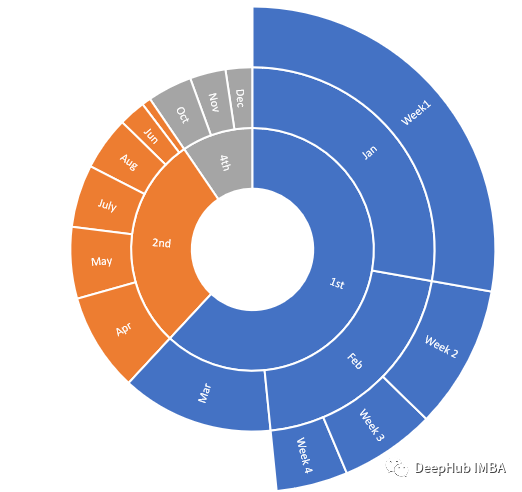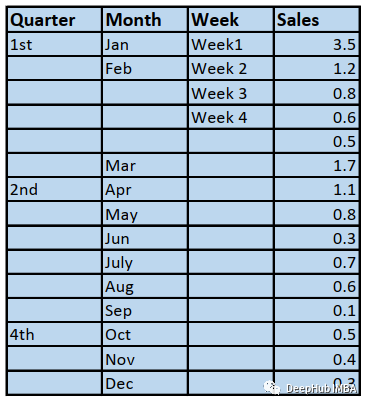`````` import plotly.express as px
df = px.data.tips()
``````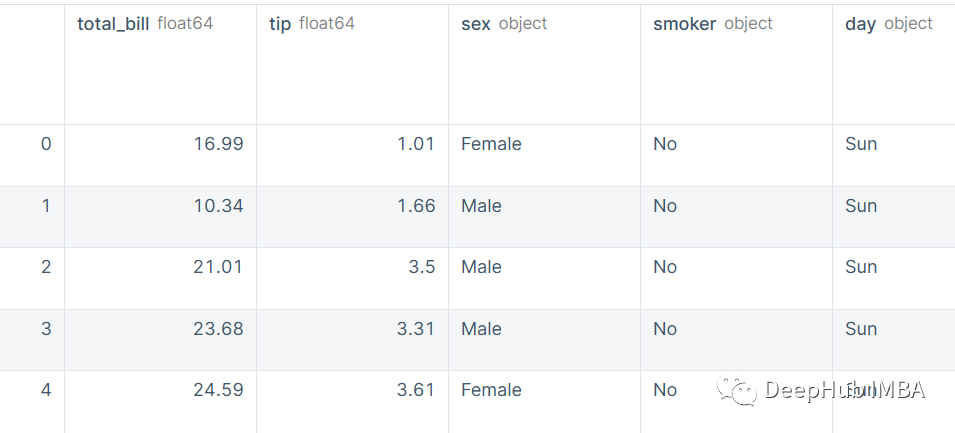`````` fig = px.sunburst(df, path=['sex', 'day', 'time'], values='total_bill', color='time')
fig.show()
``````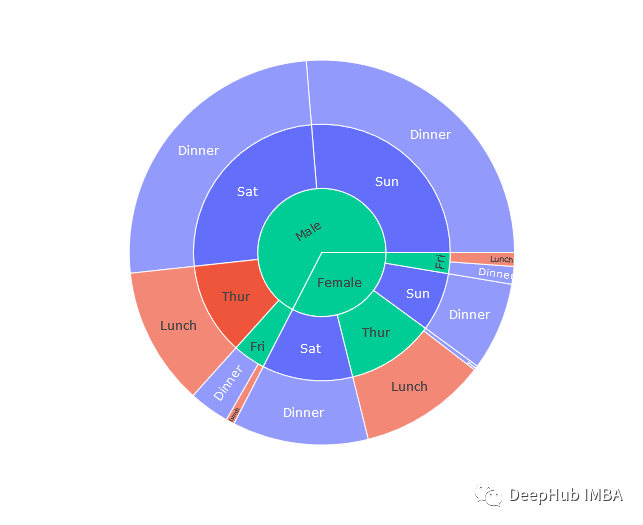sunburst类的path属性提供了层次结构，其中性别位于层次结构的顶部，然后是日期和时间。

## 10、词云（Word Cloud）

`````` import pandas as pd
``````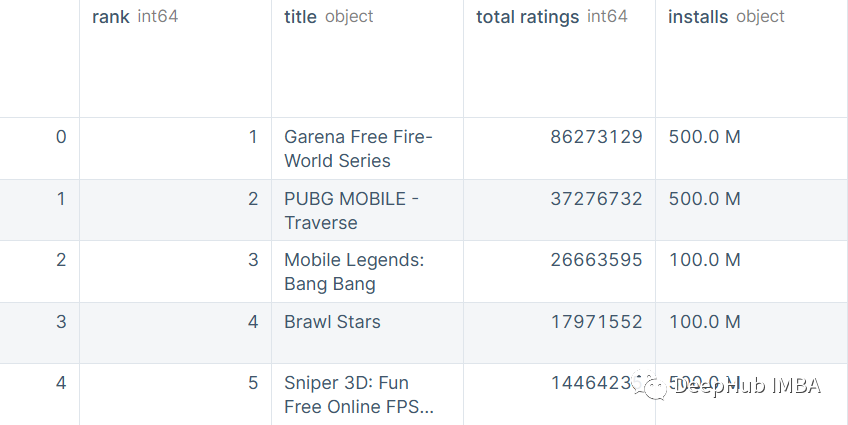`````` data.category.value_counts()
``````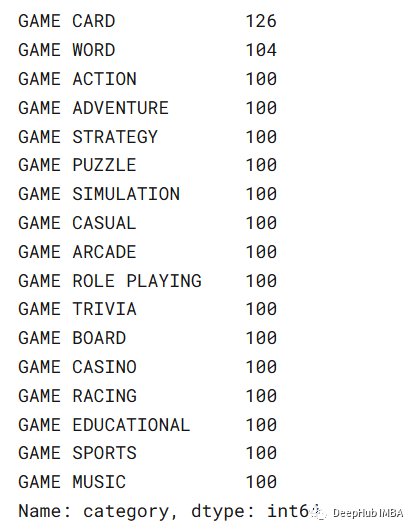`````` #importing the module from wordcloud library
from wordcloud import WordCloud
import matplotlib.pyplot as plt

#creating a text from the category  column by taking only the 2nd part of the category.
text = " ".join(cat.split() for cat in data.category)

#generating the cloud
word_cloud = WordCloud(collocations = False, background_color = 'black').generate(text)
plt.imshow(word_cloud, interpolation='bilinear')
plt.axis("off")
plt.show()
``````## 总结

https://plotly.com/python/parallel-coordinates-plot/

https://pandas.pydata.org/docs/reference/api/pandas.DataFrame.plot.hexbin.html

Hintze, V. P. A Box Plot-Density Trace Synergism. Am. Stat, (52), 181 (Open Access Journal).

seaborn.pointplot — seaborn 0.12.1 documentation (pydata.org)

seaborn.swarmplot — seaborn 0.12.1 documentation (pydata.org)

Create a sunburst chart in Office — Microsoft Support

Sunburst charts in Python (plotly.com)

### “10个实用的数据可视化的图表总结”的评论:

##### 关于作者##### Deephub